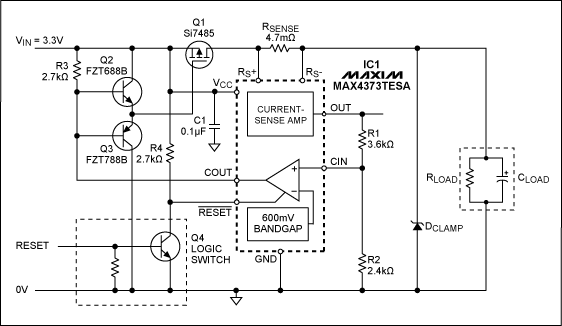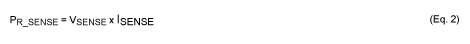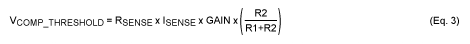# Simple, Latched Overcurrent Fault Detector Has Fast Response Time

### Abstract

The circuit introduced here is a simple, fast-responding overcurrent detector designed to protect low-voltage applications. Unlike dedicated hot-swap controllers that impose a long start-up delay initiated by undervoltage, this circuit provides protection only 150µs after the input supply rises above 2.7V. Additionally, a limited gate voltage on an external p-channel switch provides a measure of in-rush current limiting during power up.

### Overview

Figure 1 shows the complete circuit of the latched overcurrent fault detector. After power is applied, the comparator output COUT is close to zero volts. The noninverting buffer formed by Q2 and Q3 ensures that the gate of Q1, a very low on-resistance, low-threshold, p-channel power MOSFET, is fully enhanced. The resulting current into the load is measured by a high-side current-sense amplifier, which converts the small voltage across the current-sense resistor RSENSE into a scaled, ground-referenced output voltage at the OUT pin. That voltage, proportional to the load current, is further scaled at the input of a latched, noninverting comparator.

When the load current exceeds the threshold voltage at the junction of R1 and R2, the comparator changes state, causing the output voltage to be pulled high by R3. As the gate-to-source voltage falls below the gate threshold, the p-channel MOSFET is shutdown. The noninverting buffer Q2-Q3 ensures ample charging and discharging current into, and out of, the Q1 gate, resulting in fast switching.Figure 1. An integrated current-sense amplifier, latched comparator, and reference form a fast-responding, low-voltage, overcurrent-protection circuit.

### Component Selection

Controller
The MAX4373 is the controller selected for a fast-responding, current-latching, current-limit detector circuit able to operate from +3.3V supplies. The MAX4373 integrates all the elements required to produce such a circuit: a high common-mode differential voltage detector, a reference, and a latching comparator with active-low reset. Start-up delay is typically 500µs from the application of VCC, and propagation delay through the comparator is typically 4µs.

Current-Sense Resistor
When selecting a sense-resistor value to ensure optimum gain accuracy (typically 1% to 1.5%), the voltage drop at the rated current should be in the range 75mV to 100mV for gain ranges of +20V/V and +50V/V (T and F versions of the MAX4373). The following equations calculate the sense-resistor value and the power across it:The dynamic range of the output is also an important consideration. Center the nominal output voltage (corresponding to the operating/detecting current) at one half the supply voltage. Note that maximum VOUT is 250mV below the supply voltage at VCC. Thus, for VCC = +3.3V, nominal VOUT should be approximately 1.4V. In this example, a MAX4373 with a gain of +20V/V (T version) is suitable with a 70mV sense voltage.

For a sense current of 15A in this application, RSENSE = 4.6mΩ, producing a 70mV VSENSE, choose the nearest value of 4.7mΩ. Tolerance for the Tyco-Meggitt RL73H is ±1% (F suffix).

Threshold Current
Having set the current-detection amplifier, one should set up the comparator to provide a switching output voltage suitable for disabling the series power switch. A resistor-divider connects the current-amplifier output to the comparator positive input. For switching, the internally set threshold of 600mV nominal (580mV to 618mV) must be exceeded by the positive input of the comparator. To calculate the values of R1 and R2, use the following voltage-threshold equation:The current through R1 and R2 should be greater than 150nA and less than 500µA at the nominal output voltage of the current-sense amplifier. The comparator output sinks 1mA with a saturation voltage of 600mV (max). R3, the gate pull-up resistor, is calculated with the following equation:Power Switch
The key selection specifications for the external p-channel MOSFET are peak current, on-resistance, and gate voltage, closely followed by packaging. On-resistance should be chosen so that the voltage drop at the rated current is approximately the same as the current-sense voltage. That value produces similar levels of dissipation in the sense resistor and the MOSFET.

The Si7485DP MOSFET (from Siliconix) has a 9mΩ maximum on-resistance at VGS = -2.5V. This 20V p-channel device was selected for its operation at low input voltages. The worst-case steady-state dissipation is calculated with the following equation:At a 15A load current and a 9mΩ on-resistance, the Si7485DP operates 40°C to 50°C above the ambient temperature, so additional heat sinking is required according to the final application.

In this example, the power switch has a gate-charge specification of about 60nC. If a fast response is required, that value is beyond the drive capability of R3 and the low-power comparator output. A gate-drive buffer is therefore mandatory. As outlined above, Q2 and Q3 form a complementary emitter-follower driver that provides significant bipolar current gain into the gate of Q1. The transistors are chosen with good DC beta at medium collector currents of 500mA to 1A. Suitable choices are the Zetex FZT688B (npn) and FZT788B (pnp), packaged in SOT223 outlines. To calculate the gate response time, use the following equation:### Operation

Tolerance Build-Up
The value of the current actually sensed depends on tolerance build-up due to the following:

 Sense Resistor ±1% (TL3A) Sense Voltage Limits ±0.1 Gain Tolerance ±5.5% (max, includes gain and offset errors) Comparator Resistor Tolerance ±1% (R1 & R2) Comparator Threshold Tolerance ±3.3%

Ignoring the sense-voltage tolerance, overall current-sense tolerance is close to ±10.8%. Detailed limits can be calculated using the following equation:Using ±0.1% tolerance resistors for R1 and R2 reduces the error limits somewhat (approx ±1%), but the additional cost may not be supported by the end application.

Turn-0ff Transients
Fast response to a fault and subsequent current interruption are key requirements. However, energy remaining in the power leads' distributed inductance can produce damaging voltage spikes. Some of that energy is absorbed by distributed capacitance in the load supply, but a fast-responding overvoltage clamp may be required to protect the MAX4373 from transients of 28V or higher.

Results
A current probe monitors load current at the input terminal (VIN in Figure 1). Load current is increased until it reaches the threshold and triggers the circuit. Response time is about 2µs (Figure 2).Figure 2. Test results for the Figure 1 circuit show an approximate response time of 2µs.

A similar article was published in the October 2005 issue of Power Electronics Technology.